## Archimedian Double Mean Process

We can view double mean as a general process to find means of two input numbers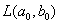using as a recurrence formula of two means.

Philips (2000) suggests Archimedean double mean process as a difference equation that involves two means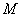and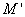with two inputs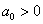and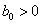, which relates to each other using the following formula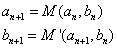Where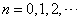Compare this difference equation formula with Gaussian Double mean process .

The Archimedean double mean process has a very nice property that the sequence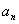and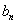will converge to a common limitwith linear convergence rate. However, the common limit is not necessarily produce means. For example, if we use arithmetic and geometric mean respectively, we will get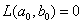which is not means or average.

Example: (Archimedean harmonic-geometric mean)

We use harmonic mean and geometric mean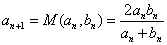and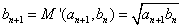Then the sequenceandwill converge to a common limitFor instance: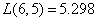,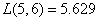,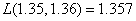,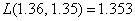Notice that this Archimedean harmonic-geometric mean is not symmetric mean, because in general,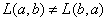.

Note that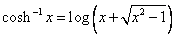Preferable reference for this tutorial is

Teknomo, Kardi (2015) Mean and Average. https:\\people.revoledu.com\kardi\tutorial\BasicMath\Average\